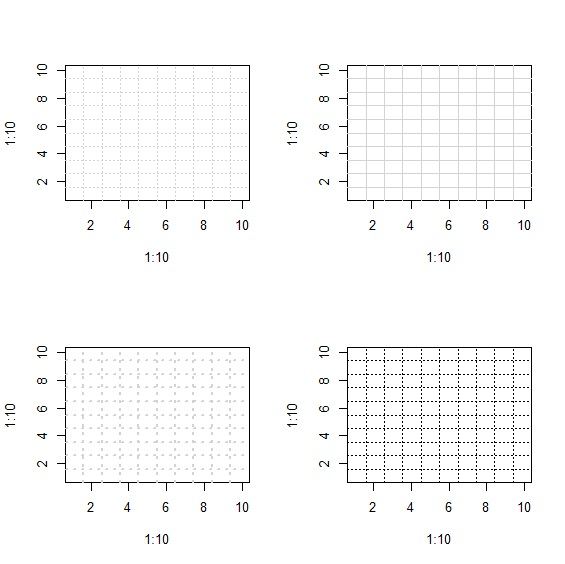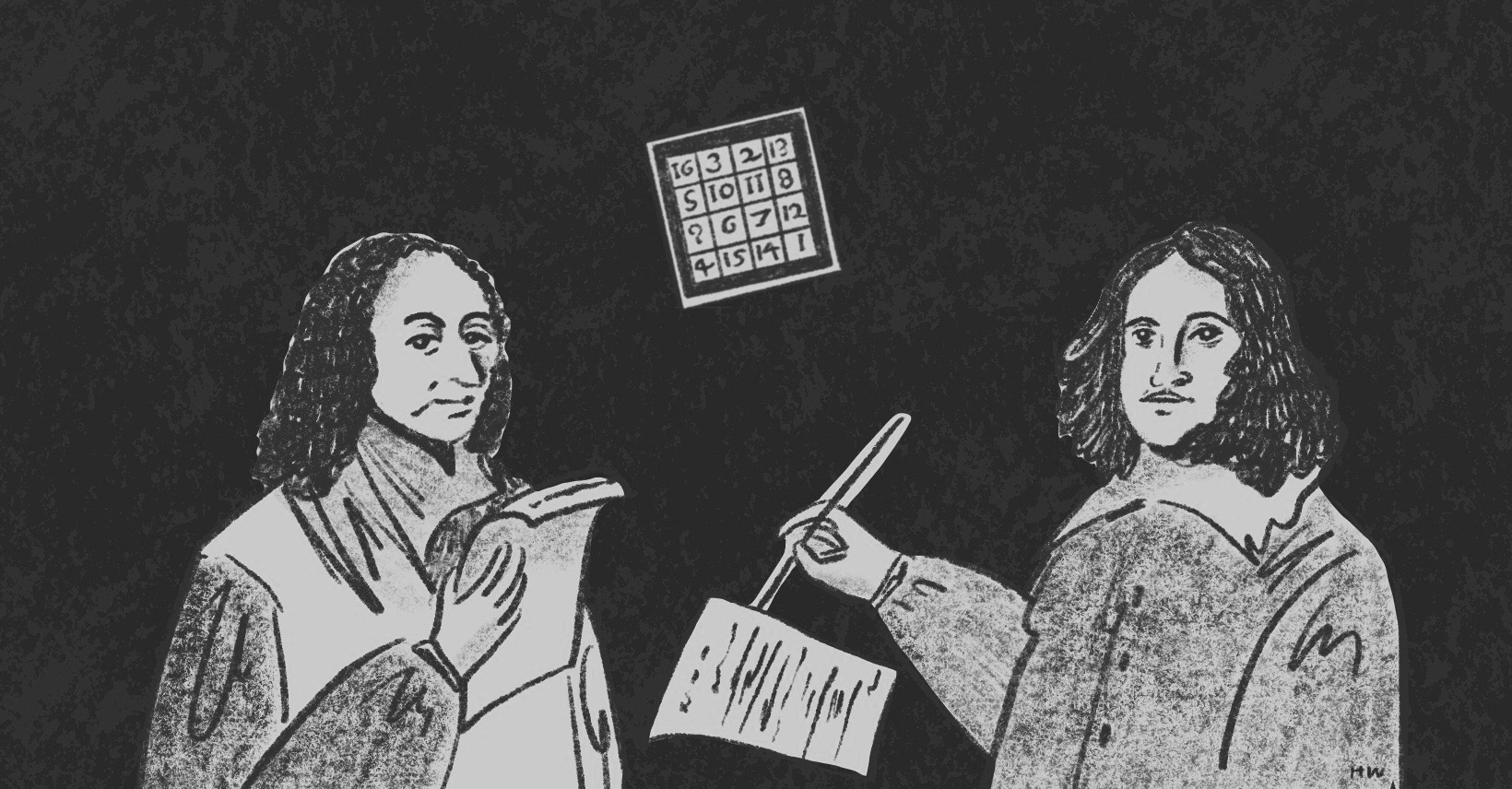×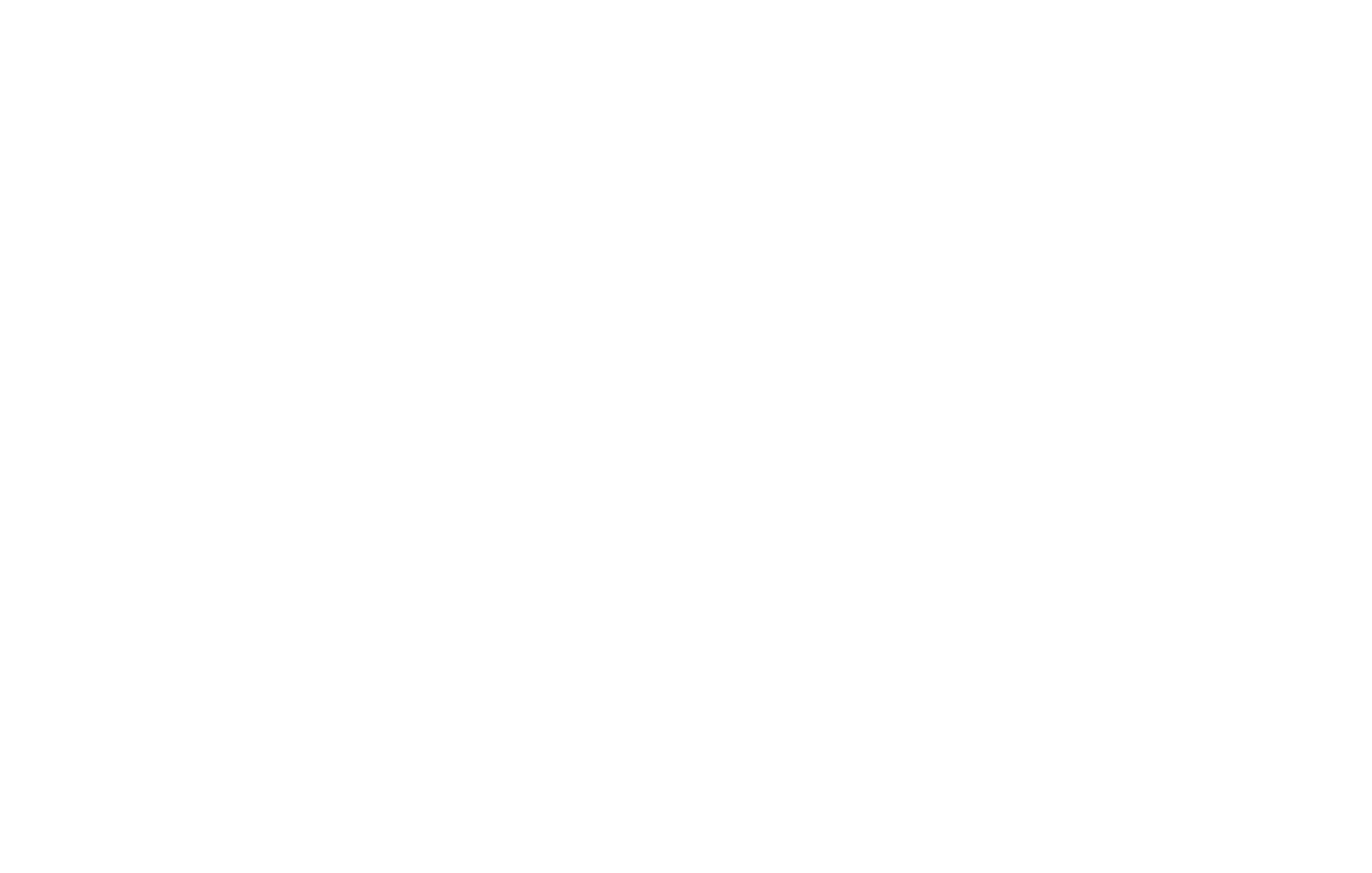資料管理 統計分析 相關資源 巨人肩膀 語法索引 關於作者

## 簡介

• type -點/線類型
• pch -資料點樣式
• cex -大小
• lty -線條樣式
• lwd -寬度
• col / bg -顏色
• xlim / ylim -軸上下限
• axes -座標軸隱藏顯示
• main, sub, xlab, ylab -標題
• ann -標題隱藏顯示
• bty -框線樣式
• frame.plot -框線顯示隱藏
• line() -增加線條
• legend() -圖例
• text() -文字
• grid() -格線

## 類型

 > x<-seq(from=-pi, to=pi, by=0.5) > par(mfrow=c(2,4)) > plot(x,sin(x), type="p", main='type="p"')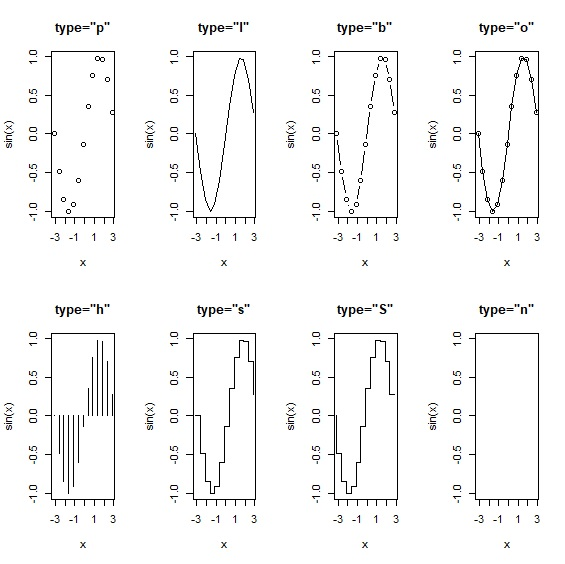## 點

pch參數可以指定pch=0~25，共計26種點的樣式，用來呈現資料點的樣式。

 > plot(1:26,pch=c(0:25), xlim=c(-1,27), ylim=c(-1,27)) > text(1:26, labels=c(0:25), pos=1, cex=0.8)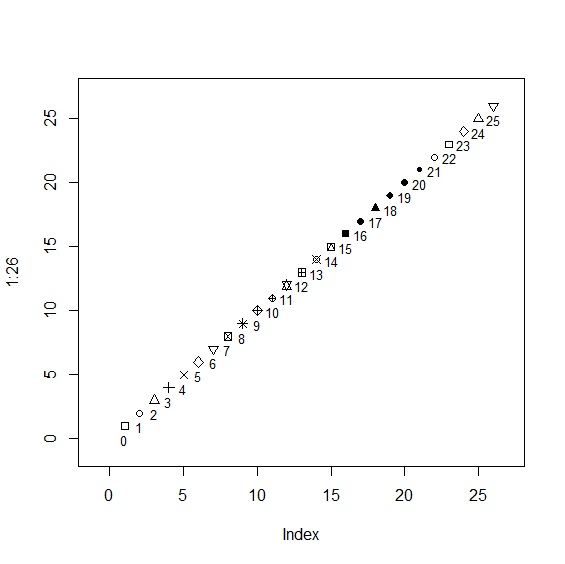## 大小

cex設定點的大小，預設值是cex=1。cex=1.5相當於放大50%，以此類推。可以配合cex.axis、cex.lab、cex.main、cex.sub設定座標軸名稱與圖表標題大小。

 > plot(1:5, 1:5, type="p", pch=17, cex=0.5, main="cex=0.5")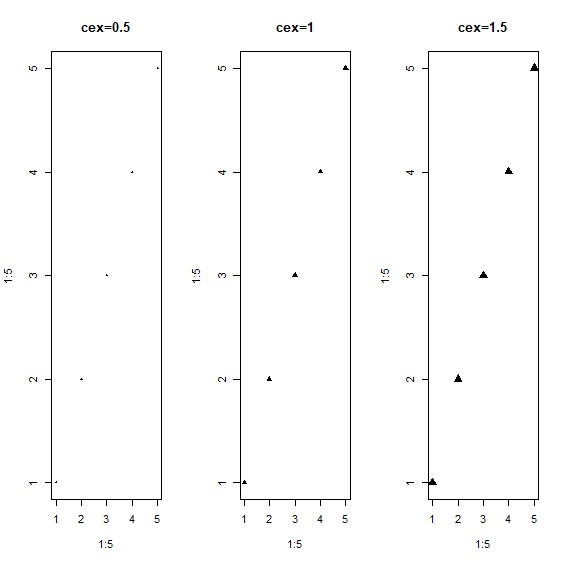## 線

lty設定線的樣式，共有solid、dashed、dotted、dotdash、longdash、twodash可選擇。

 > plot(1:5, 1:5, type="l", lty="solid", main="lty=solid")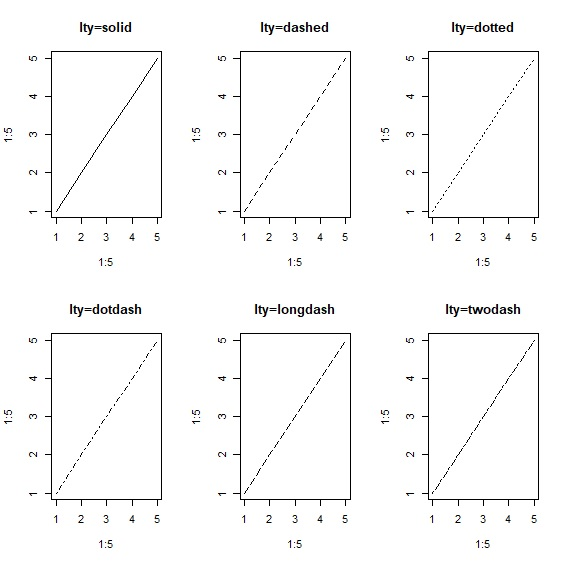## 寬度

lwd設定線條寬度，預設值是lwd=1。

 > plot(1:5, 1:5, type="l", lwd=1, main="lwd=1")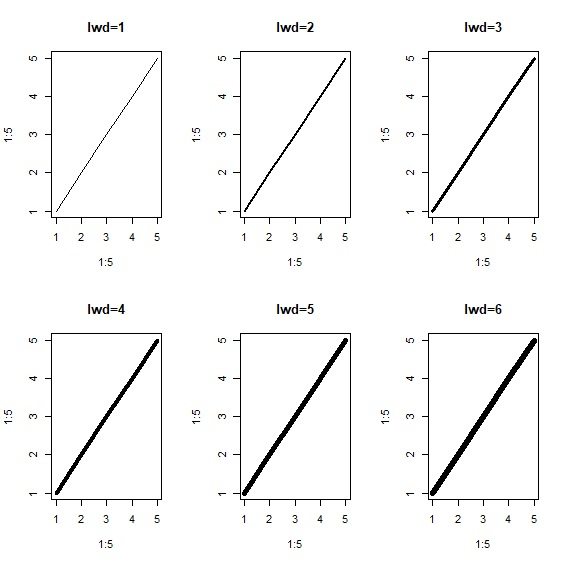## 顏色

col與bg用來設定資料點、線條的顏色。配合pch=21~25的樣式，可以設定bg背景色。配合col.axis、col.lab、col.main、col.sub等參數可設定座標軸與圖表標題顏色。

 > plot(1:5, 1:5, type="p", pch=25, col="black", bg="yellow")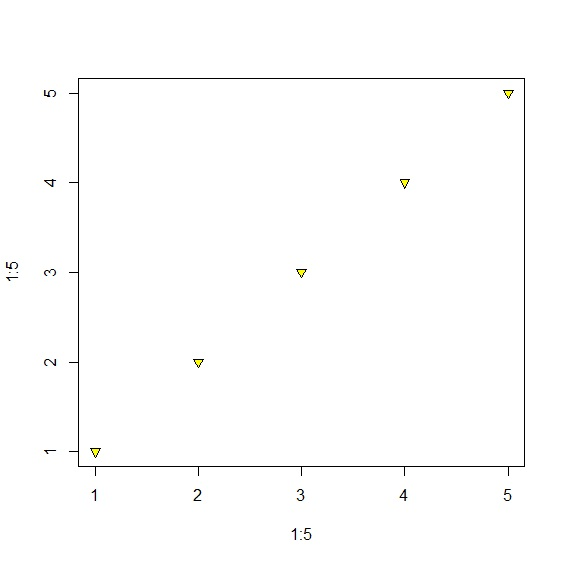## 座標軸

### 邊界

xlim與ylim設定x、y座標軸的上下界。

 > plot(x, sin(x), xlim=c(-5,5), ylim=c(-2,2)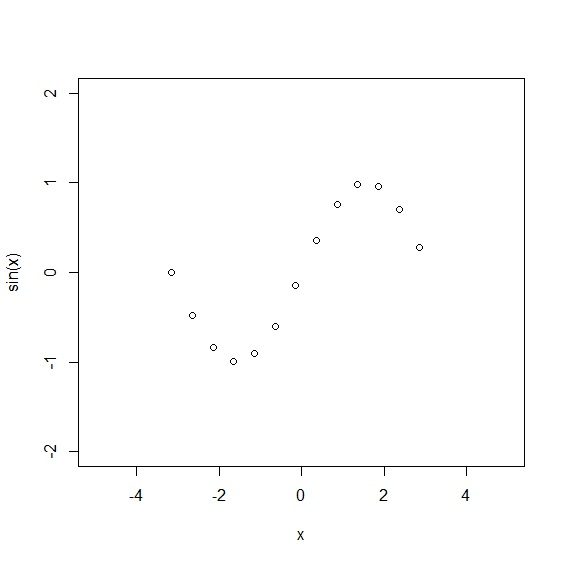### 顯示/隱藏

axes設定座標軸顯示或隱藏。當axes=TRUE時座標軸顯示，反之aexs=FALSE時隱藏座標軸。必須注意的是，axes只針對座標軸，當座標軸隱藏的時候，座標軸的名稱仍然存在。axes=FALSE與ann=FALSE一同使用時可以顯示沒有任何座標軸與文字的乾淨圖案。

 > plot(x, sin(x), axes=TRUE, main="axes=T") > plot(x, sin(x), axes=FALSE, main="axes=F")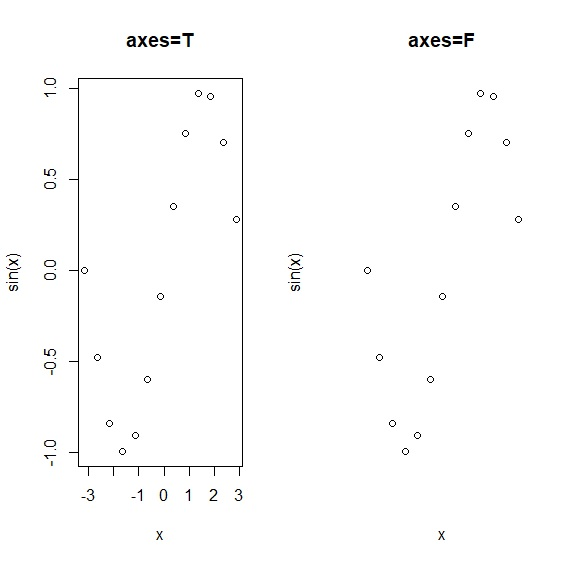## 標題

main、sub、xlab、ylab分別設定圖表的主標、副標、x軸名稱與y軸名稱。ann則設定標題是否顯示，ann=TRUE時顯示圖表標題與、x軸名稱、y軸名稱，反之不顯示。axes=FALSE與ann=FALSE一同使用時可以顯示沒有任何座標軸與文字的乾淨圖案。

 > plot(x, sin(x), main="Sine Function") > plot(x, sin(x), sub="Sine Function") > plot(x, sin(x), xlab="X-axis") > plot(x, sin(x), ylab="Y-axis") > plot(x, sin(x), ann=TRUE, main="ann=T") > plot(x, sin(x), ann=FALSE, main="ann=F")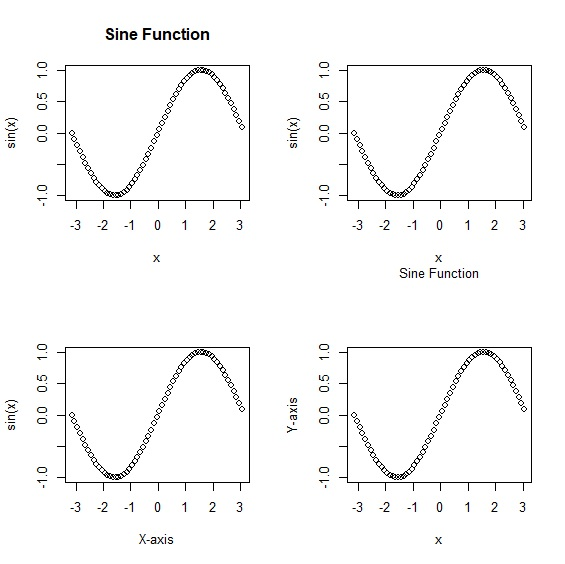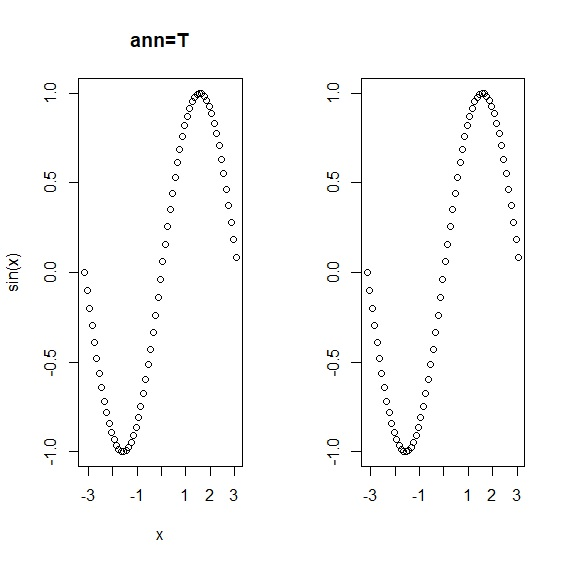## 框線

bty設定圖形輸出的外框，共有6種型態。frame.plot則是邏輯值，當frame.plot=TRUE時，圖表會加上外框線；反之則只顯示座標軸。

 > x<-seq(from=-pi, to=pi, by=0.5) > par(mfrow=c(2,3)) > plot(x,sin(x), bty="o", main='bty="o"') > par(mfrow=c(1,2)) > plot(x, sin(x), frame.plot=TRUE) > plot(x, sin(x), frame.plot=FALSE)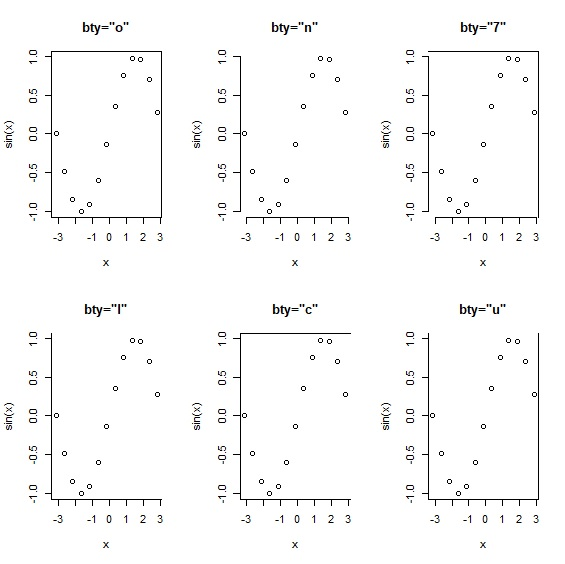## 多重線條

lines()指令可以在原圖上增加作圖，創造出疊圖效果。

 > x<-seq(from=-pi, to=pi, by=0.5) > plot(x, sin(x), type="l", col="blue") > lines(x, cos(x), col="red")") > lines(x, -sin(x), col="darkgreen") > lines(x, -cos(x), col="violet")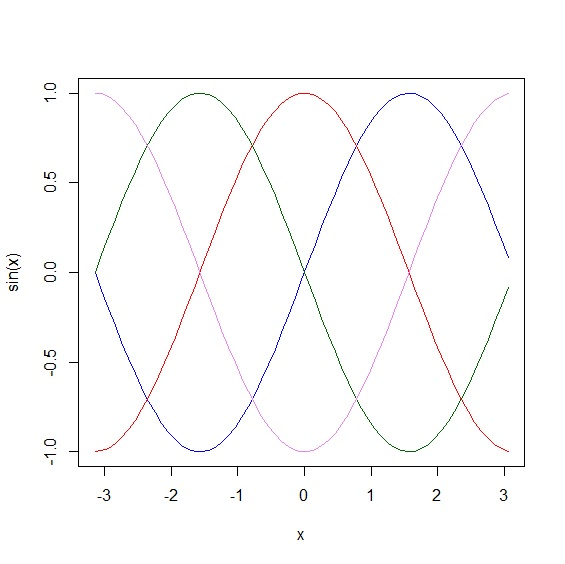## 圖例

legend()指令處理圖例，也可以搭配lty、cex、bg等參數，設定圖例的樣式。

 > legend("bottomleft", c("sin", "cos", "sec", "csc"), fill=c("blue", "red", "darkgreen", "violet"))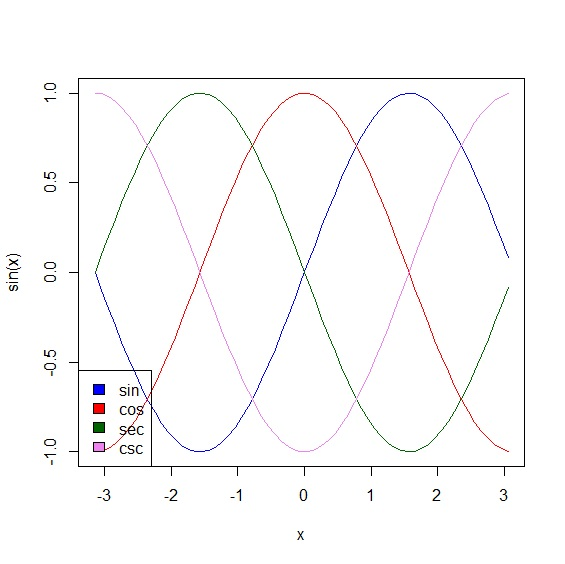## 文字

text()可以在圖上增加文字。

### 位置

 > text(x=1,y=10, labels=c("文字內容")) 

### 字體

font參數設定粗體、斜體、斜粗體。搭配font.main、font.sub、font.lab、font.axis可以設定圖標題與座標軸的字型。

 > plot(1:11, 1:11, type="n") > text(x=2,y=2, labels=c("1=plain"), font=1) > text(x=4,y=4, labels=c("2=bold"), font=2) > text(x=6,y=6, labels=c("3=italic"), font=3) > text(x=8,y=8, labels=c("4=bold-italic"), font=4) > text(x=10,y=10, labels=c("5=symbol"), font=5)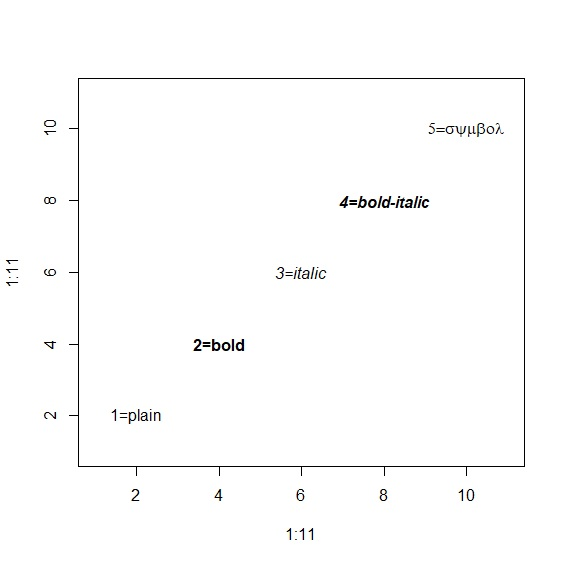### 標籤與對齊

labels以及pos分別設定文字標籤與對齊方向。pos參數從1~4分別是靠下、靠左、靠上、靠右對齊。

 > plot(1:10, 1:10, type="n") > text(x=1,y=1, labels=c("bottomleft"), pos=4, col="blue") > text(x=1,y=10, labels=c("topleft"), pos=4, col="red") > text(x=10,y=10, labels=c("topright"), pos=2, col="darkgreen") > text(x=10,y=1, labels=c("bottomright"), pos=2, col="purple") > text(x=5.5,y=6, labels=c("below"), pos=1, col="black") > text(x=5.5,y=6, labels=c("left"), pos=2, col="black") > text(x=5.5,y=6, labels=c("above"), pos=3, col="black") > text(x=5.5,y=6, labels=c("right"), pos=4, col="black")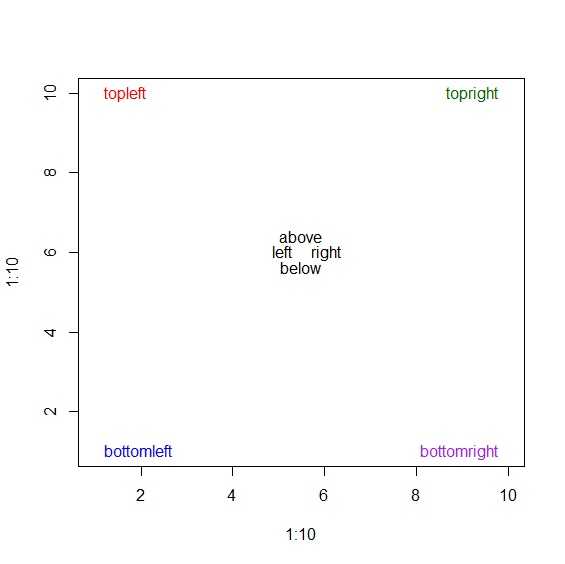## 格線

grid()用來增加圖的格線。

 > plot(1:10,1:10, type="n") > grid(10,10) > grid(10,10, lty="solid") > grid(10,10, lwd=2) > grid(10,10, col="black")